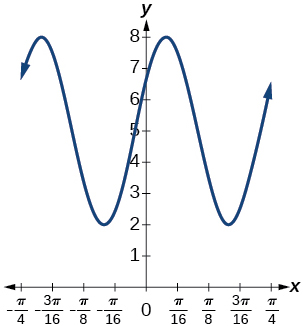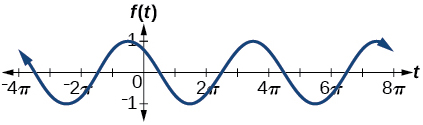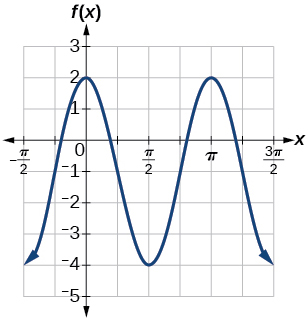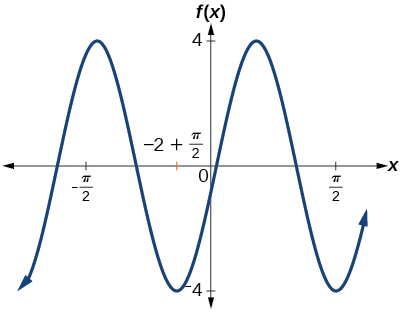# 6.1E: Exercises

$$\newcommand{\vecs}{\overset { \rightharpoonup} {\mathbf{#1}} }$$ $$\newcommand{\vecd}{\overset{-\!-\!\rightharpoonup}{\vphantom{a}\smash {#1}}}$$$$\newcommand{\id}{\mathrm{id}}$$ $$\newcommand{\Span}{\mathrm{span}}$$ $$\newcommand{\kernel}{\mathrm{null}\,}$$ $$\newcommand{\range}{\mathrm{range}\,}$$ $$\newcommand{\RealPart}{\mathrm{Re}}$$ $$\newcommand{\ImaginaryPart}{\mathrm{Im}}$$ $$\newcommand{\Argument}{\mathrm{Arg}}$$ $$\newcommand{\norm}{\| #1 \|}$$ $$\newcommand{\inner}{\langle #1, #2 \rangle}$$ $$\newcommand{\Span}{\mathrm{span}}$$ $$\newcommand{\id}{\mathrm{id}}$$ $$\newcommand{\Span}{\mathrm{span}}$$ $$\newcommand{\kernel}{\mathrm{null}\,}$$ $$\newcommand{\range}{\mathrm{range}\,}$$ $$\newcommand{\RealPart}{\mathrm{Re}}$$ $$\newcommand{\ImaginaryPart}{\mathrm{Im}}$$ $$\newcommand{\Argument}{\mathrm{Arg}}$$ $$\newcommand{\norm}{\| #1 \|}$$ $$\newcommand{\inner}{\langle #1, #2 \rangle}$$ $$\newcommand{\Span}{\mathrm{span}}$$$$\newcommand{\AA}{\unicode[.8,0]{x212B}}$$

## 6.1: Graphs of the Sine and Cosine Functions

In the chapter on Trigonometric Functions, we examined trigonometric functions such as the sine function. In this section, we will interpret and create graphs of sine and cosine functions

### Verbal

1) Why are the sine and cosine functions called periodic functions?

The sine and cosine functions have the property that $$f(x+P)=f(x)$$ for a certain $$P$$. This means that the function values repeat for every $$P$$ units on the $$x$$-axis.

2) How does the graph of $$y=\sin x$$ compare with the graph of $$y=\cos x$$? Explain how you could horizontally translate the graph of $$y=\sin x$$ to obtain $$y=\cos x$$.

3) For the equation $$A \cos(Bx+C)+D$$$,$what constants affect the range of the function and how do they affect the range?

The absolute value of the constant $$A$$ (amplitude) increases the total range and the constant $$D$$ (vertical shift) shifts the graph vertically.

4) How does the range of a translated sine function relate to the equation $$y=A \sin(Bx+C)+D$$$?$

5) How can the unit circle be used to construct the graph of $$f(t)=\sin t$$?

At the point where the terminal side of $$t$$ intersects the unit circle, you can determine that the $$\sin t$$ equals the $$y$$-coordinate of the point.

### Graphical

For the following exercises, graph two full periods of each function and state the amplitude, period, and midline. State the maximum and minimum $$y$$-values and their corresponding $$x$$-values on one period for $$x>0$$. Round answers to two decimal places if necessary.

6) $$f(x)=2\sin x$$

7) $$f(x)=\dfrac{2}{3}\cos x$$amplitude: $$\dfrac{2}{3}$$$;$period: $$2\pi$$$;$midline: $$y=0$$$;$maximum: $$y=\dfrac{2}{3}$$ occurs at $$x=0$$$;$minimum: $$y=-\dfrac{2}{3}$$ occurs at $$x=\pi$$$;$for one period, the graph starts at $$0$$ and ends at $$2\pi$$.

8) $$f(x)=-3\sin x$$

Exercise $$\PageIndex{9}$$

$$f(x)=4\sin x$$amplitude: $$4$$; period: $$2\pi$$$;$midline: $$y=0$$$;$maximum $$y=4$$ occurs at $$x=\dfrac{\pi }{2}$$$;$minimum: $$y=-4$$ occurs at $$x=\dfrac{3\pi }{2}$$$;$one full period occurs from $$x=0$$ to $$x=2\pi$$

10) $$f(x)=2\cos x$$

Exercise $$\PageIndex{11}$$

$$f(x)=\cos (2x)$$amplitude: $$1$$; period: $$\pi$$$;$midline:

12) $$f(x)=2 \sin \left(\dfrac{1}{2}x\right)$$

13) $$f(x)=4 \cos(\pi x)$$amplitude: $$4$$; period: $$2$$; midline: $$y=0$$$;$maximum: $$y=4$$ occurs at $$x=0$$$;$minimum: $$y=-4$$ occurs at $$x=1$$

14) $$f(x)=3 \cos\left(\dfrac{6}{5}x\right)$$

15) $$y=3 \sin(8(x+4))+5$$amplitude: $$3$$; period: $$\dfrac{\pi }{2}$$$;$ midline: $$y=5$$$;$ maximum: $$y=8$$ occurs at $$x=0.12$$$;$ minimum: $$y=2$$ occurs at $$x=0.516$$$;$ horizontal shift: $$-4$$$;$ vertical translation $$5$$; one period occurs from $$x=0$$ to $$x=\dfrac{\pi }{4}$$

16) $$y=2 \sin(3x-21)+4$$

17) $$y=5 \sin(5x+20)-2$$amplitude: $$5$$; period:$$\dfrac{2\pi }{5}$$$;$ midline: $$y=-2$$$;$ maximum: $$y=3$$ occurs at $$x=0.08$$$;$ minimum: $$y=-7$$ occurs at $$x=0.71$$$;$ phase shift: $$-4$$$;$ vertical translation: $$-2$$$;$ one full period can be graphed on $$x=0$$ to$$x=\dfrac{2\pi }{5}$$

For the following exercises, graph one full period of each function, starting at $$x=0$$. For each function, state the amplitude, period, and midline. State the maximum and minimum $$y$$-values and their corresponding $$x$$-values on one period for $$x>0$$. State the phase shift and vertical translation, if applicable. Round answers to two decimal places if necessary.

18) $$f(t)=2\sin \left(t-\dfrac{5\pi}{6} \right)$$

19) $$f(t)=-\cos \left(t+\dfrac{\pi}{3} \right)+1$$amplitude: $$1$$; period: $$2\pi$$$;$ midline: $$y=1$$$;$ maximum: $$y=2$$ occurs at $$x=2.09$$$;$ maximum: $$y=2$$ occurs at $$t=2.09$$$;$ minimum: $$y=0$$ occurs at $$t=5.24$$$;$ phase shift: $$-\dfrac{\pi}{3}$$$;$ vertical translation: $$1$$; one full period is from $$t=0$$ to $$t=2\pi$$

20) $$f(t)=4\cos \left(2\left (t+\dfrac{\pi}{4} \right ) \right)-3$$

21) $$f(t)=-\sin \left (\dfrac{1}{2}t+\dfrac{5\pi}{3} \right )$$amplitude: $$1$$; period: $$4\pi$$$;$ midline: $$y=0$$$;$ maximum: $$y=1$$ occurs at $$t=11.52$$$;$ minimum: $$y=-1$$ occurs at $$t=5.24$$$;$ phase shift: $$-\dfrac{10\pi}{3}$$$;$ vertical shift: $$0$$

22) $$f(x)=4\sin \left (\dfrac{\pi}{2}(x-3) \right )+7$$

Exercise $$\PageIndex{23}$$

Determine the amplitude, midline, period, and an equation involving the sine function for the graph shown in Figure below.amplitude: $$2$$; midline: $$y=-3$$ ;   period: $$4$$; equation: $$f(x)=2\sin \left (\dfrac{\pi}{2}x \right )-3$$

24) Determine the amplitude, period, midline, and an equation involving cosine for the graph shown in the Figure below.25) Determine the amplitude, period, midline, and an equation involving cosine for the graph shown in the Figure below.amplitude: $$2$$; period: $$5$$; midline: $$y=3$$$;$ equation: $$f(x)=-2\cos \left (\dfrac{2\pi}{5}x \right )+3$$

26) Determine the amplitude, period, midline, and an equation involving sine for the graph shown in the Figure below.27) Determine the amplitude, period, midline, and an equation involving cosine for the graph shown in the Figure below.amplitude: $$4$$; period: $$2$$; midline: $$y=0$$$;$ equation: $$f(x)=-4\cos \left (\pi \left (x-\dfrac{\pi}{2} \right ) \right )$$

28) Determine the amplitude, period, midline, and an equation involving sine for the graph shown in the Figure below.29) Determine the amplitude, period, midline, and an equation involving cosine for the graph shown in the Figure below.amplitude: $$2$$; period: $$2$$; midline $$y=1$$$;$ equation: $$f(x)=2\cos \left (\pi x \right )+1$$

30) Determine the amplitude, period, midline, and an equation involving sine for the graph shown in the Figure below.

### Algebraic

For the following exercises, let $$f(x)=\sin x$$$.$

31) On $$[0,2\pi )$$$,$ solve $$f(x)=0$$$.$

32) On $$[0,2\pi )$$ , solve $$f(x)=\dfrac{1}{2}$$.

$$\dfrac{\pi }{6}$$, $$\dfrac{5\pi }{6}$$

33) Evaluate $$f \left( \dfrac{\pi }{2} \right)$$$.$

34) On $$[0,2\pi)$$, $$f(x)=\dfrac{\sqrt{2}}{2}$$

$$\dfrac{\pi }{4}$$, $$\dfrac{3\pi }{4}$$

35) On $$[0,2\pi )$$$,$ the maximum value(s) of the function occur(s) at what $$x$$-value(s)?

36) On $$[0,2\pi )$$$,$ the minimum value(s) of the function occur(s) at what $$x$$-value(s)?

$$\dfrac{3\pi }{2}$$

37) Show that $$f(-x) = -f(x)$$$.$ This means that $$f(x)=\sin x$$ is an odd function and possesses symmetry with respect to ________________.

For the following exercises, let $$f(x)=\cos x$$

38) On $$[0,2\pi )$$$,$ solve the equation $$f(x)=\cos x=0$$

$$\dfrac{\pi }{2}$$, $$\dfrac{3\pi }{2}$$

39) On $$[0,2\pi )$$$,$ solve $$f(x)=\dfrac{1}{2}$$.

40) On $$[0,2\pi )$$$,$ find the $$x$$-intercepts of $$f(x)=\cos x$$.

$$\dfrac{\pi }{2}$$, $$\dfrac{3\pi }{2}$$

41) On $$[0,2\pi )$$$,$ find the $$x$$-values at which the function has a maximum or minimum value.

42) On $$[0,2\pi )$$$,$ solve the equation $$f(x)=\dfrac{\sqrt{3}}{2}$$$.$

$$\dfrac{\pi }{6}$$, $$\dfrac{11\pi }{6}$$

### Technology

43) Graph $$h(x)=x+\sin x$$ on $$[0,2\pi ]$$$.$ Explain why the graph appears as it does.

44) Graph $$h(x)=x+\sin x$$ on $$[-100,100]$$$.$ Did the graph appear as predicted in the previous exercise?

The graph appears linear. The linear functions dominate the shape of the graph for large values of $$x$$.45) Graph $$f(x)=x\sin x$$ on $$[0,2\pi ]$$ and verbalize how the graph varies from the graph of $$f(x)=x\sin x$$.

46) Graph $$f(x)=x\sin x$$ on the window $$[-10,10]$$ and explain what the graph shows.

The graph is symmetric with respect to the $$y$$-axis and there is no amplitude because the function is not periodic.47) Graph $$f(x)=\dfrac{\sin x}{x}$$ on the window $$[-5\pi , 5\pi ]$$ and explain what the graph shows.

### Real-World Applications

48) A Ferris wheel is $$25$$ meters in diameter and boarded from a platform that is $$1$$ meter above the ground. The six o’clock position on the Ferris wheel is level with the loading platform. The wheel completes $$1$$ full revolution in $$10$$ minutes. The function $$h(t)$$ gives a person’s height in meters above the ground $$t$$ minutes after the wheel begins to turn.

1. Find the amplitude, midline, and period of $$h(t)$$.
2. Find a formula for the height function $$h(t)$$.
3. How high off the ground is a person after $$5$$ minutes?
1. Amplitude: $$12.5$$; period: $$10$$; midline: $$y=13.5$$
2. $$h(t)=12.5\sin\left ( \dfrac{\pi}{5}(t-2.5) \right )+13.5$$
3. $$26$$ ft